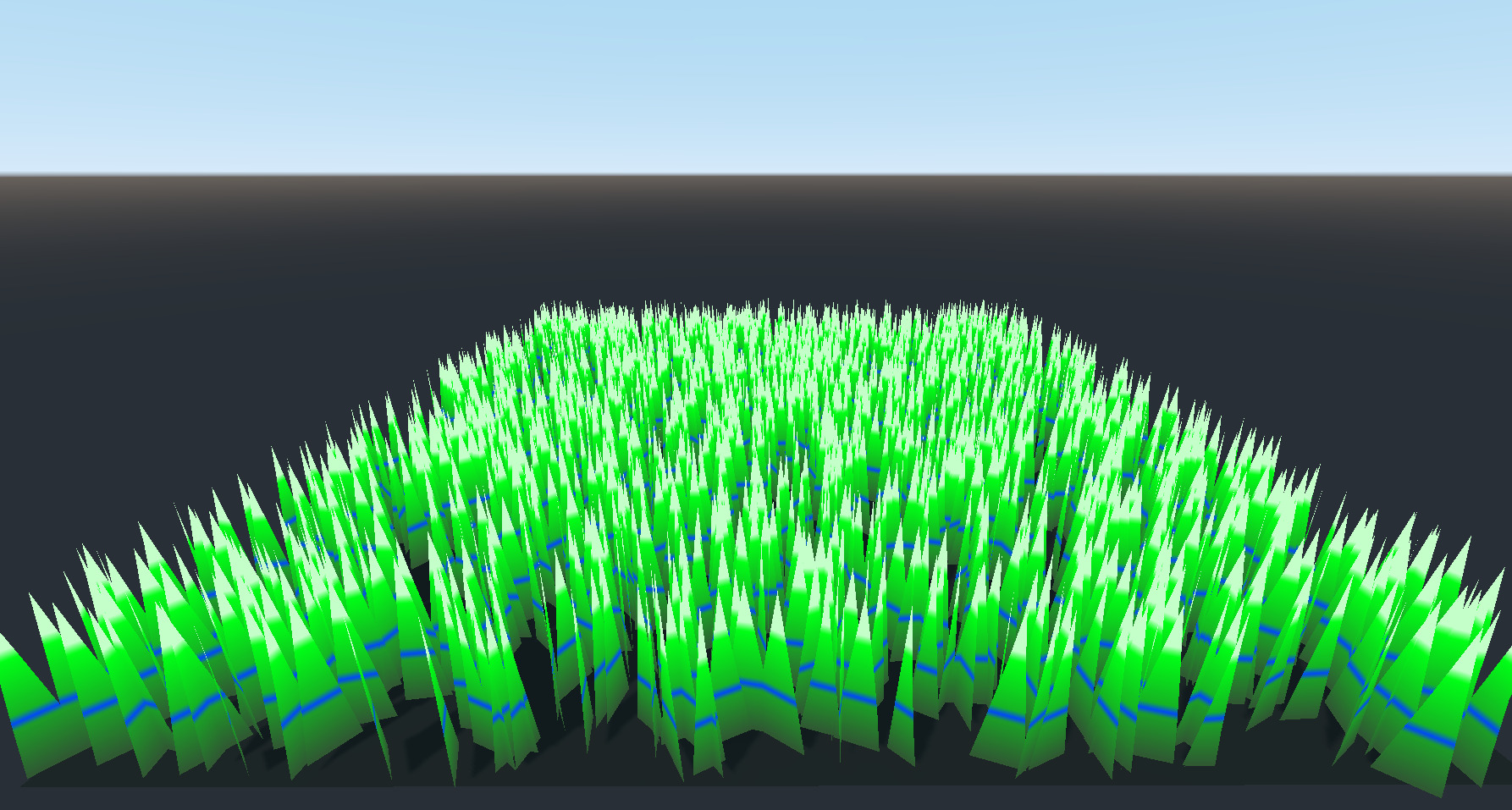This is a tweak to GDQuest “Stylized grass with wind and deformation” shader (link)

I tweaked the shader to better handle using a gradient texture for “Color Ramp”. As seen in the preview, different colours can be set just like any regular colour gradient.

Changes:

• Added “gradient_blade_length” uniform, which is used to determine the length of the grass for UV calculations
• Modified the colour sampling to use the Y position of the object vertex coordinate instead of the UVs, this fixes the calculation for models where the UVs are not set properly. Minimal math is used for this calculation, retaining performance considerations from original shader

All credit for original shader goes to GDQuest! <3

``````shader_type spatial;

uniform float wind_speed = 0.2;
uniform float wind_strength = 2.0;
// How big, in world space, is the noise texture
// wind will tile every wind_texture_tile_size
uniform float wind_texture_tile_size = 20.0;
uniform float wind_vertical_strength = 0.3;
uniform vec2 wind_horizontal_direction = vec2(1.0,0.5);

uniform sampler2D color_ramp : hint_black_albedo;
// we need a tiling noise here!
uniform sampler2D wind_noise : hint_black;

// it is used to calculate a relational UV for the grass blade on the y-axis
// tweaking this will affect how the gradient displays for better or worse

uniform vec3 character_position;
uniform sampler2D character_distance_falloff_curve : hint_black_albedo;
uniform float character_push_strength = 1.0;

varying float debug_wind;
varying float y_coord;

void vertex() {

vec3 world_vert = (WORLD_MATRIX * vec4(VERTEX, 1.0)).xyz;
y_coord = VERTEX.y / gradient_blade_length; // use the local vertex position. This assumed the model file has the bottom at y=0
vec2 normalized_wind_direction = normalize(wind_horizontal_direction);
vec2 world_uv = world_vert.xz / wind_texture_tile_size + normalized_wind_direction * TIME * wind_speed;
// we displace only the top part of the mesh
// note that this means that the mesh needs to have UV in a way that the bottom of UV space
// is at the top of the mesh
float displacement_affect = (1.0 - UV.y);
float wind_noise_intensity = (textureLod(wind_noise, world_uv , 0.0).r - 0.5);

// We convert the direction of the wind into vertex space from world space
// if we used it directly in vertex space, rotated blades of grass wouldn't behave properly
vec2 vert_space_horizontal_dir = (inverse(WORLD_MATRIX) * vec4(wind_horizontal_direction, 0.0,0.0)).xy;
vert_space_horizontal_dir = normalize(vert_space_horizontal_dir);

vec3 bump_wind = vec3(
wind_noise_intensity * vert_space_horizontal_dir.x,
1.0 - wind_noise_intensity,
wind_noise_intensity * vert_space_horizontal_dir.y
);
normalize(bump_wind);
bump_wind *= vec3(
wind_strength,
wind_vertical_strength,
wind_strength
);
VERTEX += bump_wind * displacement_affect;

// At the moment the blades are pushed away in a perfectly circular manner.
// We could distort the distance to the character based on a noise, to break a bit the
// circular shape. We could distort the falloff by sampling in a noise based on the xz coordinates.

vec3 dir_to_character = character_position - WORLD_MATRIX.xyz;
// uncomment the following line to have a horizontal only character push
//	dir_to_character.y = 0.0;
float distance_to_character = length(dir_to_character);
float falloff = 1.0 - smoothstep(0.0, 1.0, distance_to_character/character_radius);
// Because we operate in vertex space, we need to convert the direction to the character
// in vertex space. Otherwise, it wouldn't work for rotated blades of grass.
// comment the next line to observe how the blades are not all facing away from the character.
dir_to_character = (inverse(WORLD_MATRIX) * vec4(dir_to_character, 0.0)).xyz;
dir_to_character = normalize(dir_to_character);

// sample the curve based on how far we are from the character, in normalized coordinates
float falloff_curve = texture(character_distance_falloff_curve, vec2(falloff)).x;
// direction to character is inverted because we want to point away from it
VERTEX += normalize(-dir_to_character) * falloff_curve * character_push_strength * displacement_affect;

}

void fragment() {
ALBEDO = texture(color_ramp, vec2(y_coord, 0)).rgb; // use the new y-coord instead of UV
}``````
###### Tags
3d, deformation, grass, tweak, windThe shader code and all code snippets in this post are under MIT license and can be used freely. Images and videos, and assets depicted in those, do not fall under this license. For more info, see our License terms.

## More from QueenOfSquiggles

### QoS Style World Space Blue Noise Dither Effect